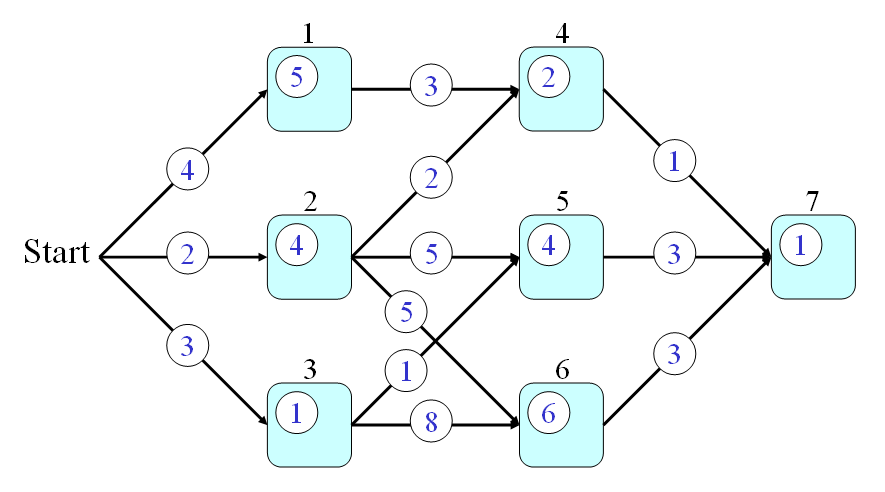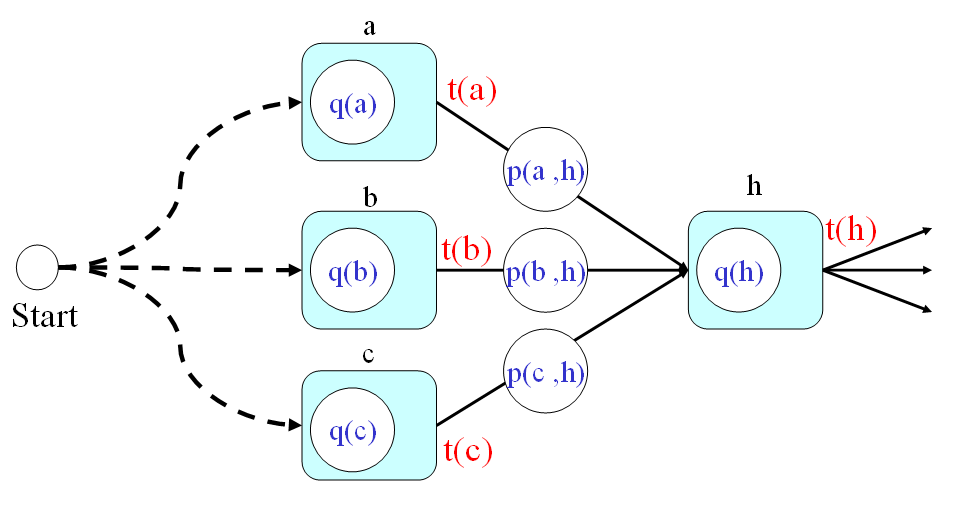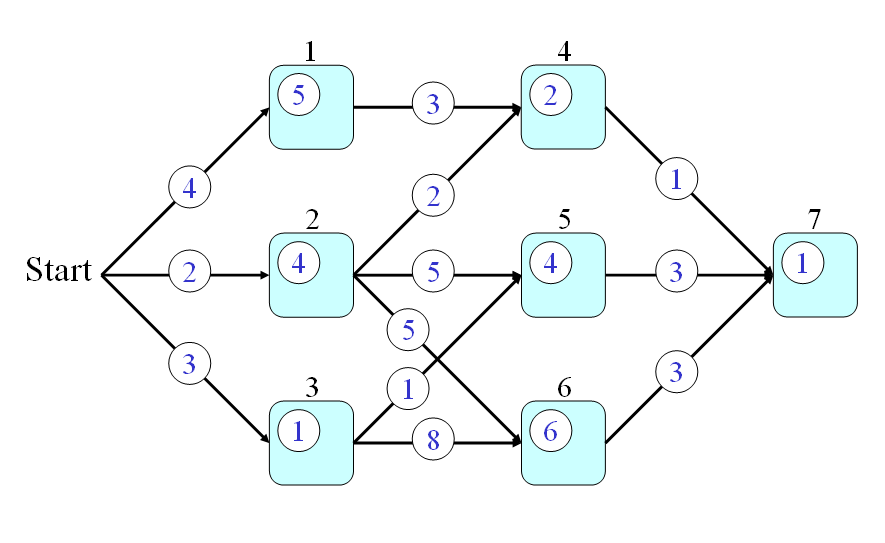8-1 Introduction to Dynamic Programming (嚙踝蕭嚙踝蕭嚙踝蕭嚙踝蕭嚙踝蕭嚙踝蕭)

[chinese][all]

Dynamic programming (DP) is an effective method for finding an optimum solution to a multi-stage decision problem, as long as the solution can be defined recursively. DP roots in the principle of optimality proposed by Richard Bellman:

An optimal policy has the property that whatever the initial state and the initial decisions are, the remaining decisions must constitute an optimal policy with regard to the state resulting from the first decision.

In terms of path finding problems, the principle of optimality states that any partial path of the optimum path is also an optimum path given the starting and ending nodes. This is an obvious principle that can be proved by contradiction. In practice, a number of seemingly unrelated applications can be effectively solved by DP.

To employ the procedure of DP to solve a problem, usually we need to specify the following items:

1. Define the optimum-value function.
2. Derive the recursive formula for the optimum-value function, together with its initial condition.
3. Specify the answer of the problem in term of the optimum-value function.

We shall give an example of DP for a path finding problem shown in the following graph:In the above graph, we assume that:

• Every node is a city and we use q(a) to represent the time required to go through node a, where a is in {1, 2, 3, 4, 5, 6, 7}.
• Every link is a route connecting two city. We use p(a, b) to represent the time required to go from nodes a to b, where a and b are in {1, 2, 3, 4, 5, 6, 7}.

From the start point, how can we find a path that requires the minimum time to pass through node 7? This is a typical problem of DP which can be solved systematically and efficiently by the following three steps:

1. First of all, we can define the optimum-value function t(h) as the minimum time from the start point to node h (including the time for passing node h).
2. Secondly, the optimum-value function should satisfy the following recursive formula:
t(h) = min{t(a)+p(a,h), t(b)+p(b,h), t(c)+p(c,h)} + q(h)

In the above equation, we assume the fan-ins of node h are a, b, and c. Please refer to the next figure.And the initial condition is t(0)=0 where 0 is the start node.
3. And finally, the answer to the original problem should be t(7). By using the recursion, we can have the following steps for computing the time required by the optimum path:
1. t(0) = 0
2. t(1) = t(0)+4+5 = 9
3. t(2) = t(0)+2+4 = 6
4. t(3) = t(0)+3+1 = 4
5. t(4) = min(9+3, 6+2)+2 = 10
6. t(5) = min(6+5, 4+1)+4 = 9
7. t(6) = min(6+5, 4+8)+6 = 17
8. t(7) = min(10+1, 9+3, 17+3)+1 = 12
The value of the optimum-value function is shown as the red number in each node in the following figure:Hint
You can open a new window by clicking the above figure, and then click the newly opened window to see each step of the recursion by DP.

The above formulation of DP is usually referred to as the forward DP. On the other hand, we can define the formula for backward DP in a similar manner:
1. Firstly, the optimum-value function s(h) is defined as the minimum time from a node h to the end node (including the time for passing the end node but excluding the time for passing node h.)
2. Secondly, the optimum-value function should satisfy the following recursive formula:
s(h) = q(h) + min{p(h,a)+s(a), p(h,b)+s(b), p(h,c)+s(c)}
where a, b, c are the fan-out of node h. The initial condition is s(7) = q(7).
3. Finally, the answer to the original problem is s(0). By using the recursion, we can have the following steps for computing the time required by the optimum path:
1. s(7) = 1
2. s(6) = q(6)+3+s(7) = 6+3+1 = 10
3. s(5) = q(5)+3+s(7) = 4+3+1 = 8
4. s(4) = q(4)+1+s(7) = 2+1+1 = 4
5. s(3) = q(3)+min{1+s(5), 8+s(6)} = 1 + min{9, 18} = 10
6. s(2) = q(2)+min{2+s(4), 5+s(5), 5+s(6)} = 4 + min{6, 13, 15} = 10
7. s(1) = q(1)+3+s(4) = 5 + 3 + 4 = 12
8. s(0) = min{4+s(1), 2+s(2), 3+s(3)} = min(16, 12, 13} = 12
The answer obtained from backward DP is the same as that of the forward DP.

The efficiency of DP is based on the fact that we can use some previous results iteratively. Some common characteristics of DP are summarized as follows:

• After finding the optimum fan-in path of a given node, it would be better to keep this information in the data structure in order to back track the optimum path at the end of DP.
• DP usually only gives the optimum path. If we want to find the second-best path, we need to invoke another version of DP to find the n-best path, which is beyond the scope of this tutorial.

Data Clustering and Pattern Recognition (資料分群與樣式辨認)